## Python 数据可视化分析¶

• 单变量可视化的常用方法
• 多变量可视化的常用方法
• t-SNE

### 数据集¶

In :
import warnings

import matplotlib.pyplot as plt
import numpy as np
import pandas as pd
import seaborn as sns

sns.set()
warnings.filterwarnings("ignore")


In :
df = pd.read_csv("../../data/telecom_churn.csv")

In :
df.head()

Out:
State Account length Area code International plan Voice mail plan Number vmail messages Total day minutes Total day calls Total day charge Total eve minutes Total eve calls Total eve charge Total night minutes Total night calls Total night charge Total intl minutes Total intl calls Total intl charge Customer service calls Churn
0 KS 128 415 No Yes 25 265.1 110 45.07 197.4 99 16.78 244.7 91 11.01 10.0 3 2.70 1 False
1 OH 107 415 No Yes 26 161.6 123 27.47 195.5 103 16.62 254.4 103 11.45 13.7 3 3.70 1 False
2 NJ 137 415 No No 0 243.4 114 41.38 121.2 110 10.30 162.6 104 7.32 12.2 5 3.29 0 False
3 OH 84 408 Yes No 0 299.4 71 50.90 61.9 88 5.26 196.9 89 8.86 6.6 7 1.78 2 False
4 OK 75 415 Yes No 0 166.7 113 28.34 148.3 122 12.61 186.9 121 8.41 10.1 3 2.73 3 False

### 单变量可视化¶

#### 直方图和密度图¶

In :
features = ["Total day minutes", "Total intl calls"]
df[features].hist(figsize=(10, 4))

Out:
array([[<matplotlib.axes._subplots.AxesSubplot object at 0x7f59f62f72b0>,
<matplotlib.axes._subplots.AxesSubplot object at 0x7f59f62bcb00>]],
dtype=object)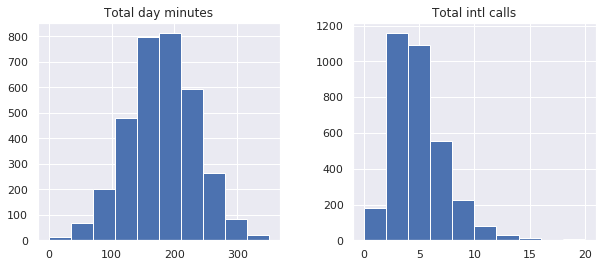In :
df[features].plot(
kind="density",
subplots=True,
layout=(1, 2),
sharex=False,
figsize=(10, 4),
legend=False,
title=features,
)

Out:
array([[<matplotlib.axes._subplots.AxesSubplot object at 0x7f59f399e0f0>,
<matplotlib.axes._subplots.AxesSubplot object at 0x7f59f395a9e8>]],
dtype=object)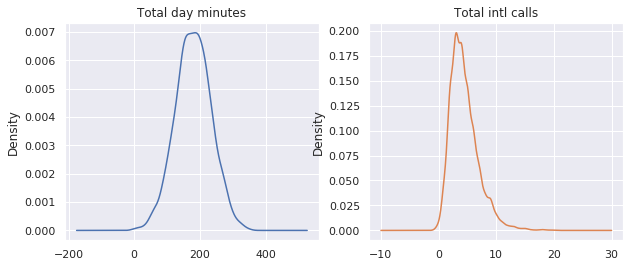In :
sns.distplot(df["Total intl calls"])

Out:
<matplotlib.axes._subplots.AxesSubplot at 0x7f59f695ce80>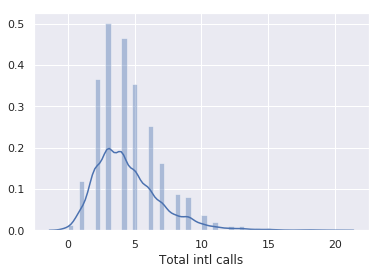#### 箱型图¶

• 箱子显示了分布的四分位距，它的长度由 $25th \, （\text{Q1，下四分位数}）$ 和 $75th \, （\text{Q3，上四分位数}）$ 决定，箱中的水平线表示中位数 （$50\%$）。
• 须是从箱子处延伸出来的线，它们表示数据点的总体散布，具体而言，是位于区间 $（\text{Q1} - 1.5 \cdot \text{IQR}, \text{Q3} + 1.5 \cdot \text{IQR}）$的数据点，其中 $\text{IQR} = \text{Q3} - \text{Q1}$，也就是四分位距。
• 离群值是须之外的数据点，它们作为单独的数据点，沿着中轴绘制。

In :
sns.boxplot(x="Total intl calls", data=df)

Out:
<matplotlib.axes._subplots.AxesSubplot at 0x7f59e9c3cc50>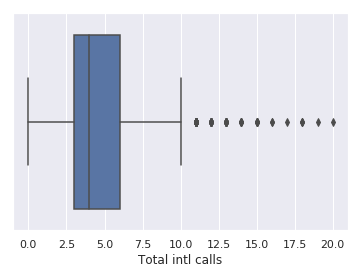#### 提琴形图¶

In :
_, axes = plt.subplots(1, 2, sharey=True, figsize=(6, 4))
sns.boxplot(data=df["Total intl calls"], ax=axes)
sns.violinplot(data=df["Total intl calls"], ax=axes)

Out:
<matplotlib.axes._subplots.AxesSubplot at 0x7f59e9b6ba20>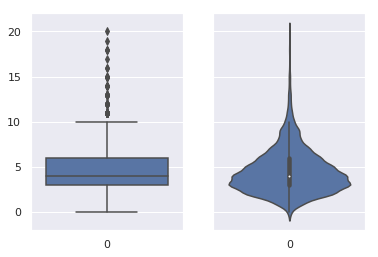#### 数据描述¶

In :
df[features].describe()

Out:
Total day minutes Total intl calls
count 3333.000000 3333.000000
mean 179.775098 4.479448
std 54.467389 2.461214
min 0.000000 0.000000
25% 143.700000 3.000000
50% 179.400000 4.000000
75% 216.400000 6.000000
max 350.800000 20.000000

describe() 的输出基本上是自解释性的，25%，50% 和 75% 是相应的百分数

#### 频率表¶

In :
df["Churn"].value_counts()

Out:
False    2850
True      483
Name: Churn, dtype: int64

#### 条形图¶

In :
_, axes = plt.subplots(nrows=1, ncols=2, figsize=(12, 4))

sns.countplot(x="Churn", data=df, ax=axes)
sns.countplot(x="Customer service calls", data=df, ax=axes)

Out:
<matplotlib.axes._subplots.AxesSubplot at 0x7f59e9a97400>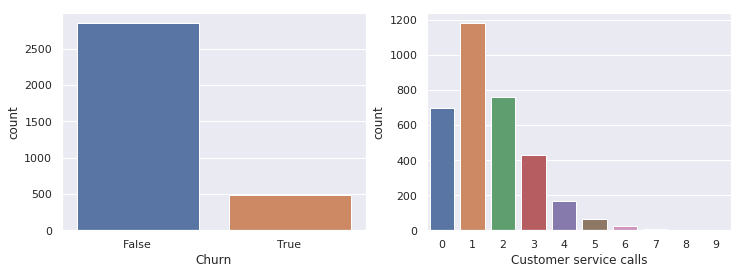• 直方图适合查看数值变量的分布，而条形图用于查看类别特征。
• 直方图的 X 轴是数值；条形图的 X 轴可能是任何类型，如数字、字符串、布尔值。
• 直方图的 X 轴是一个笛卡尔坐标轴；条形图的顺序则没有事先定义。

### 多变量可视化¶

#### 相关矩阵¶

In :
# 丢弃非数值变量
numerical = list(
set(df.columns)
- set(
[
"State",
"International plan",
"Voice mail plan",
"Area code",
"Churn",
"Customer service calls",
]
)
)
# 计算和绘图
corr_matrix = df[numerical].corr()
sns.heatmap(corr_matrix)

Out:
<matplotlib.axes._subplots.AxesSubplot at 0x7f59e97352e8>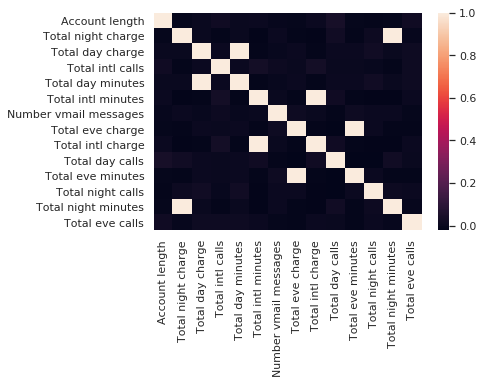In :
numerical = list(
set(numerical)
- set(
[
"Total day charge",
"Total eve charge",
"Total night charge",
"Total intl charge",
]
)
)


#### 散点图¶

In :
plt.scatter(df["Total day minutes"], df["Total night minutes"])

Out:
<matplotlib.collections.PathCollection at 0x7f59e86a3e48>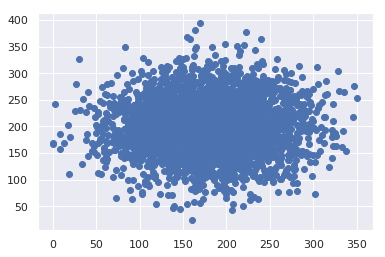seaborn 库的 方法在绘制散点图的同时会绘制两张直方图，某些情形下它们可能会更有用。

In :
sns.jointplot(x="Total day minutes", y="Total night minutes", data=df, kind="scatter")

Out:
<seaborn.axisgrid.JointGrid at 0x7f59e8658dd8>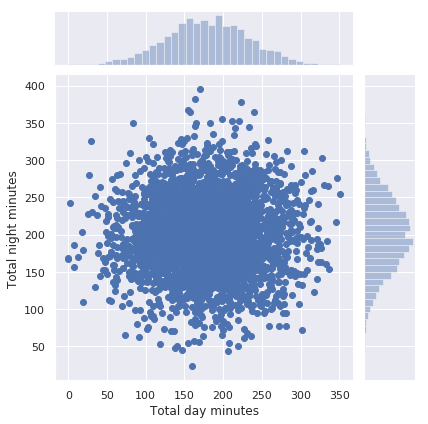jointplot() 方法还可以绘制平滑过的散点直方图。

In :
sns.jointplot(
"Total day minutes", "Total night minutes", data=df, kind="kde", color="g"
)

Out:
<seaborn.axisgrid.JointGrid at 0x7f59e852f5c0>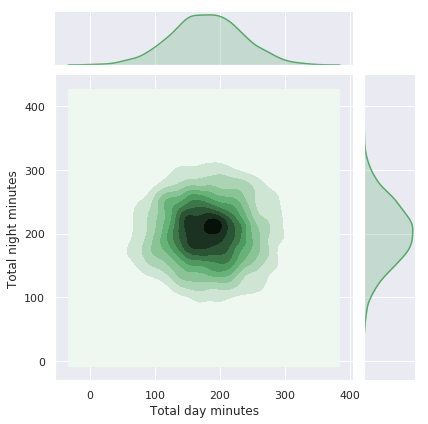#### 散点图矩阵¶

In :
%config InlineBackend.figure_format = 'png'
sns.pairplot(df[numerical])

Out:
<seaborn.axisgrid.PairGrid at 0x7f59e8389240>